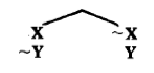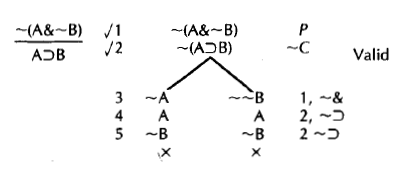# 8.4: The Complete Rules for the Connectives

All we have left to do is to state the rules for the remaining connectives. And this further development involves nothing very different from what we have done already. The reasoning that enables us to figure out the new rules is just like the reasoning we went over in explaining the rules for disjunction, negated disjunction, and negated conjunction.

To see how to state the rules generally, let us start with one we have already seen in action:

Rule V: If a disjunction of the form XvY appears as the entire sentence at a point on a tree, write the branchat the bottom of every open path on which XvY appears.

Notice that I have stated the general rule using bold face 'X' and 'Y' instead of sentence letters 'A' and 'B'. This is because I want to be able to apply the rule to more complicated sentences, which result if we substitute compound sentences for the 'X' and the 'Y'. Don't worry about this for now. We will work on this complication in the next chapter.
We continue with a statement of the other two rules which we have already seen in action:

Rule ~v: If a negated disjunction of the form ~(XvY) appears as the entire sentence at a point on a tree, write the stack

~X
~Y

at the bottom of every open path on which ~(XvY) appears.

Rule -&: If a negated conjunction of the form ~(X&Y) appears as the entire sentence at a point on a tree, write the branchat the bottom of every open path on which ~(X&Y) appears.

Did you try your hand at exercise 8-2? If not, or if you did and had trouble, try again, now that you have seen how I have given a general statement of the rules we have already worked with. Try to figure out the new rules for the other connectives by thinking through what, for example, you would need to do to a tree to make true a conjunction which appears on a tree, or a conditional, or a negated conditional.

In order to make a conjunction true on a path we have to make both. conjuncts true. This is the only minimally sufficient way of making the conjunction true. So the rule for conjunction is

Rule &: If a conjunction of the form X&Y appears as the entire sentence at a point on a tree, write the stack

X
Y

at the bottom of every open path on which X&Y occurs.

Now let's try the conditional. How can we make a conditional true? If we make the antecedent false, that does it right there. Making the antecedent false is minimally sufficient for making the conditional true. Similarly, making just the consequent true is minimally sufficient for making the conditional true. Clearly, we need a branch, each fork of which has one of the minimally sufficient ways of making the conditional true. One fork of the branch will have the negation of the antecedent, and the other fork will have the consequent:

Rule⊃: If a sentence of the form X⊃Y appears as the entire sentence at a point on a tree, write the branchat the bottom of every open path on which X⊃Y occurs.

What about negated conditionals? A negated conditional produces a stack as do conjunctions and negated disjunctions. A negated conditional can be made true only by making its antecedent true and its consequent false at the same time. So our rule for negated conditionals reads ,

Rule ~⊃: If a sentence of the form ~(X⊃Y) appears as the entire sentence at a point on a tree, write the stack

X
~Y

at the bottom of every open path on which ~(X⊃Y) appears.

The rule for the biconditional is just a bit more complicated. You can't make the biconditional X≡Y true just by making one component true or false. You have to assign a truth value to two components to make it true. So far, the rule for X≡Y is looking like that for X&Y. But there are two independent ways of making X≡Y true. We can make it true by making both components true, and we can make it true by making both components false. Finally, these are all the minimally sufficient ways of making X≡Y true. So we will have to make a branch, each fork of which will have two sentences:

Rule ≡: If a biconditional of the form X≡Y appears as the entire sentence at a point on a tree, write the branchat the bottom of every open path on which X≡Y appears.

Note that the rule ≡ looks different from all the previous rules. Each previous rule instructed you to branch or stack, but not both. The rule ≡ requires both branching and stacking. We need a branch with two forks, and each fork has a stack of two sentences. Only thus do we get all the minimally sufficient ways of making a biconditional true.

The reasoning behind the rule for the negated biconditional looks very similar to that for the conditional. A negated biconditional is true just in case the biconditional itself is false. Under what conditions is a biconditional false? It's false when the components have different truth values. This can happen in two ways: The biconditional is false when the first component is true and the second component is false, and it is false when the first component is false and the second component is true. This gives us the rule Rule

Rule ~≡: If a negated biconditional of the form ~(X≡Y) appears as the entire sentence at a point on a tree, write the branchat the bottom of every open path on which ~(X≡Y) appears.

As with the conditional, we need two branches, each with a stack of two sentences. Only in this way will we get all the minimally sufficient ways of making a negated biconditional true.

We need one last truth tree rule. Consider the following argument and the tree for testing its validity:How did I get the branch on the right to close? We can look at this in two equally correct ways. We can note that '~~B' is the negation of '~B'. Thus we have an inconsistent pair of sentences on the same branch. This is what I hope occurred to you when you met double negations in the previous exercises in this chapter. I can no more make '~B' and '~~B' both true than I can make 'B' and '~B' both true. So the branch closes. Also, we can observe that '~~B' is logically equivalent to 'B'. Clearly, the one will be true if and only if the other is true. So we can make '~~B' true by making 'B' true. We could rewrite the right branch on the above tree as follows:

~~B 1, ~&
B 3, ~~
A 2, ~⊃
~B 2, ~⊃
x

We will formalize this move in a further rule:

Rule ~~: If the sentence ~~X appears as the entire sentence at a point on a tree, write X at the bottom of every open path on which ~~X appears.

These nine rules for the connectives tell you what to do when working on a sentence which appears as the entire sentence at a point on a tree. The examples should give you a pretty good idea of how to go about applying these rules. But let's review the basic ideas:

A truth tree works by looking for an assignment of truth values to sentence letters which makes all of the tree's original sentences true.

We will see in the next chapter that a truth tree can be used for other things in addition to checking arguments for validity. But so far we have studied only validity checking:

To use the truth tree method to test an argument for validity, list, as the initial sentences of a tree, the argument's premises and the negation of its conclusion.

You then apply the rules in this way:

1) Starting with a tree's initial sentences, you may apply the rules in any order.

2) After applying a rule to a sentence, check the sentence, to remind yourself that you do not need to work on that sentence again.

3) After working on a sentence, look to see if any paths have closed. Mark any closed paths with an ' x '.

4) Continue working on unchecked sentences until the tree has been completed, which happens when either
a) All paths have closed, or
b) No unchecked sentences are left to which you can apply a rule.

Now we need to review how you should interpret a tree once it has been completed:

An open path provides an assignment of truth values to sentence letters as follows: Assign the truth value t to each sentence letter which occurs as the entire sentence at some point along the open path. Assign the truth value f to each sentence letter whose negation occurs as the entire sentence along the open path.

In a completed tree the assignment of truth values to sentence letters provided by an open path makes all sentences along the path true. If all paths have closed, there is no assignment of truth values to sentence letters which makes all the initial sentences in the tree true.

When we use a tree to test an argument for validity, we apply this last general fact about trees, as follows:

Suppose we have a completed tree whose initial sentences are an argument's premises and the negation of the argument's conclusion. An open path in this tree provides an assignment of truth values to sentence letters which makes all of the initial sentences true, and so makes the argument's premises true and its conclusion false. Such an assignment is a counterexample to the argument, showing the argument to be invalid. If all of the tree's paths have closed, there is no such assignment, hence no counterexample, and the argument is valid.

We will also always use the following practical procedure:

When using the truth tree method to test an argument for validity, write next to your completed tree the word 'valid' or 'invalid' to record what the tree shows about the argument. Also, if the tree shows the argument to be invalid, write down all the counterexamples which the tree provides to the argument.

Of course, one counterexample is enough to show that an argument is invalid. But you should practice your skill at constructing trees, and as part of this practice it is useful to read off all the counterexamples a tree provides.